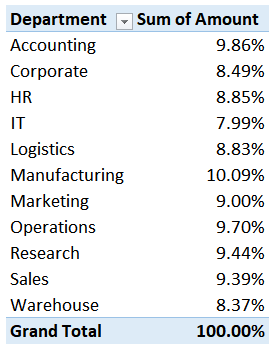## Impossible PivotTables 2 – Show Values As

This is the second post in the Impossible PivotTables series, where we are exploring Power Pivot. In the first post, we examined the limitation of calculated field references in a traditional PivotTable. In this post, we’ll address the limitation of using the Show Values As option for subsequent calculations. If we use the Show Values As option to change the way the report values are displayed, such as a percent of the grand total, we get unexpected results when we try to use those results in other calculations. To help visualize this, let’s move to an illustration and talk about the overall objective.

## Objective

Our goal is to allocate a \$10,000 corporate insurance expense to our departments. We’ve decided to allocate it based upon the relative salary of each department. Mechanically, we need to compute the percentage of each department’s salary, and then multiply the percentages by the \$10,000 corporate insurance expense.

So, we grab our monthly salary data, and begin with a traditional PivotTable. We place the Department field on rows, and we insert the Amount field into the values layout area. Then, we right-click any amount cell and select Show Values As > % of Grand Total. The resulting PT is shown below.So far, so good. This is perfect. Now, all we need to do is apply these percentages to the \$10,000 corporate expense amount. So, we crack open the calculated field dialog, and write a simple formula to multiply \$10,000 by the amount field, as shown below.When we hit OK … YIKES!!!! The results are outrageous, as shown below.What just happened here?

Each department’s allocation amount should be only a fraction of the \$10,000. And, the allocation grand total should be \$10,000. When we look at the Sum of Allocation column, the results are, well, unexpected.

When we used the Show Values As command to display the amount as a percent of the grand total, Excel changed the displayed values … but not the underlying report values. So, the Sum of Allocation column is actually displaying \$10,000 times the Amount value (not the percent of total), as shown below.And, so this becomes another situation where a traditional PT doesn’t support the type of report we are trying to build. We have another impossible PivotTable and resort to some type of workaround, such as using a helper column or writing the formulas outside the PT. But, as soon as we do that, the reporting process doesn’t feel clean or genuine. It feels a little fragile and we are worried we may have issues in the future if the report ever needs an update.

Is there good news? Yes, of course. Once again, Power Pivot will make this impossible PivotTable possible.

## Overview

We will build it in three steps:

• Load the table into the data model
• Get the basic PT going
• Write the measures

Let us begin.

Note: The steps below are presented with Excel for Windows 2016. If you are using a different version of Excel, please note that the features presented may not be available or you may need to download and install the Power Pivot Add-in.

## Load the table into the data model

First, we need to get the data table loaded into the data model. We select any cell in the Wages data table, and click the Power Pivot > Add to Data Model command.

Well, that was easy. Time to get our basic PT set up.

## Get the basic PT going

We use Excel’s Insert > PivotTable command and opt to Use this workbook’s Data Model as the source, as shown below.Then, we add the Department field on rows and the Amount field on values. The updated PT is shown below.So far so good … now we need to write a few measures.

## Write the measures

First, we need to determine the total of all wages. So, we use the Power Pivot > Measures > New Measure command and enter the desired measure name, AllWages, and the corresponding formula, as shown below.This measure uses the CALCULATE function to compute the SUM of the Wages[Amount] column for ALL rows in the Wages table.

The next measure will compute the % of Total, so, we add a new measure as shown below.This measure divides the wages for each department displayed in the report by the [AllWages] measure. This will compute the wages as a percent of the total.

And finally, we just need to do the allocation. So we create a measure named Allocation to multiply the [% of Total] measure by the corporate expense of \$10,000, as shown below.The resulting PT is shown below.We can clean it up by removing the all wages measure, and bam … another impossible PivotTable made possible with Power Pivot, as shown below.If you’d like to view the Excel file used for this post, just check out the sample file. And if you have any other fun Power Pivot tips, please share by posting a comment below.

Sample File

Posted in , ,### Jeff Lenning

I love sharing the things I've learned about Excel, and I built Excel University to help me do that. My motto is: Learn Excel. Work Faster.

### Want to learn Excel?

Our Campus Pass includes access to our entire Undergrad and Masters catalog. Gamification ensures it is the most fun you can have learning Excel :)

1.Javier on July 25, 2018 at 8:58 am

I have found a simple way. If you add a column in the original table with the formula =[@Amount]/Wages[[#Totales];[Amount]]*10000
Then PT make the sum properly ;p

BR,
Javier

•Jeff Lenning on July 25, 2018 at 9:29 am

Hi Javier,
Yes, you are exactly right! Adding a helper column as you’ve written is one workaround to this limitation, as well as writing formulas outside of the PT … thanks!
Thanks
Jeff

!
!